ChemTalk

# What is Gas Pressure? A Gaseous Definition## Core Concepts

In this article, you will learn the definition of pressure, and how to apply this definition to a sample of gas. From this, you will learn how gases exert pressure on a container, and what possible applications this definition has.

## What is Pressure – A Physical Definition

The physical definition of pressure is the total amount of force over a given area. This can be described by the equation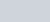, where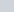is the total force, and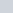is the area.Pressure is the force on an object divided by the area it is applied over.

The units of pressure are Newton per a meter squared, or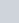## What is Pressure – A Gaseous Definition

Consider a sample of gas. In this sample of gas, there are many gas particles flying around in different directions, colliding with each other and the walls of their container. These collisions are what cause pressure. The collisions create a force on the wall of the container.

Consider what occurs when a single particle collides and bounces off of a wall at a speed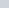. Assuming a perfectly elastic collision, this particle will accelerate by a factor of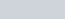. Considering Newton’s second,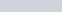, we can then see how this acceleration would exert a force on the wall.

Now, consider once more the entire sample of gas. Each particle bounces off of the walls exerting a degree of force. We can sum this force over the surface of the entire wall to get the equation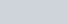.

From this equation, we can then see how a sample of gas in a container has a specific pressure.

## Measuring Pressure

When measuring the pressure of a gas in a chemistry lab, it is normally compared to atmospheric pressure. This measurement is due to everything being at atmospheric pressure. Atmospheric pressure is also relatively constant (at least when you are at similar elevations). The pressure without atmospheric pressure is referred to as gauge pressure. When gauge pressure is greater than atmospheric pressure it will be a positive value. The instruments most commonly used to measure pressure are the barometer and manometer, detailed in the linked article.

## Applications of this Definition

This definition is less of a rigorous physics definition, and more of a way to see how gas can exert a pressure. However, this particle-based definition can help us understand later how some of the specific gas laws have been derived.

## Gas Pressure Practice Problems

Problem 1

In chemistry, gas particles increase in speed when the temperature increases. Thus, if you heat up a gas, does pressure increase or decrease?

Problem 2

You have two identical amounts of gas inside containers of identical volume. One of the gases is hydrogen (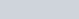) while the other gas is oxygen (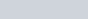). With all other physical conditions being identical, which sample of gas would exert slightly more pressure?

## Gas Pressure Practice Problem Solutions

1: Pressure increases with temperature.

2: Oxygen (it has a greater mass)